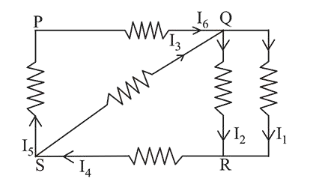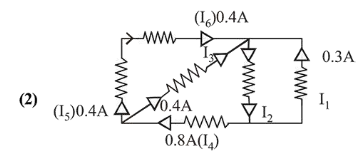# In the given circuit diagram, the currents,Question:

In the given circuit diagram, the currents, $\mathrm{I}_{1}=-0.3 \mathrm{~A}, \mathrm{I}_{4}=0.8 \mathrm{~A}$ and $\mathrm{I}_{5}=0.4 \mathrm{~A}$, are flowing as shown. The currents $\mathrm{I}_{2}, \mathrm{I}_{3}$ and $\mathrm{I}_{6}$, respectively, are :1. (1) $1.1 \mathrm{~A},-0.4 \mathrm{~A}, 0.4 \mathrm{~A}$

2. (2) $1.1 \mathrm{~A}, 0.4 \mathrm{~A}, 0.4 \mathrm{~A}$

3. (3) $0.4 \mathrm{~A}, 1.1 \mathrm{~A}, 0.4 \mathrm{~A}$

4. (4) $-0.4 \mathrm{~A}, 0.4 \mathrm{~A}, 1.1 \mathrm{~A}$

Correct Option: , 2

Solution:From $\mathrm{KCL}, I_{3}=0.8-0.4=0.4 \mathrm{~A}$

$I_{2}=0.4+0.4+0.3$

$=1.1 \mathrm{~A}$

and $\quad I_{6}=0.4 \mathrm{~A}$